# GRE Math : How to find the length of the side of an acute / obtuse triangle

## Example Questions

### Example Question #1 : How To Find The Length Of The Side Of An Acute / Obtuse Triangle

A triangle has sides 3, 5, and x. What can side x not be equal to?

6

9

3

4

9

Explanation:

This question draws from the Third Side Rule of triangles. The length of any side of a triangle must be greater than the difference between the other sides and less than the sum of the other two sides.

This means that side x must be between 2 and 8 since the difference between 5 – 3 = 2 and the sum of 3 + 5 = 8.

Choices 3, 4, and 6 all fall within the range of 2 to 8, but choice 9 does not. The answer is 9.

### Example Question #5 : Acute / Obtuse Triangles

Which of these side lengths cannot form a triangle?

7, 7, 12

120, 205, 310

6, 9, 14

25, 37, 66

5, 5, 5

25, 37, 66

Explanation:

Two sides of a triangle must add up to greater than the third side. 25, 37, 66 cannot be the lengths of the sides of a triangle as 25 + 37 < 66.

### Example Question #6 : Acute / Obtuse Triangles

The sides of a triangle are 6, 12, and an integer x. How many possible values does x have?

11

1

124

6

2

11

Explanation:

If two sides of a triangle are 6 and 12, the third must be greater than 12-6 and less than 12+6 since two sides cannot be summed to be greater than the third side in a triangle.  There are 11 possible values for x: 7, 8, 9, 10,11, 12, 13, 14, 15, 16, 17.

### Example Question #7 : Acute / Obtuse Triangles

Two sides of a triangle are 5 and 7. Which CANNOT be the length of the third side?

9

12

3

5

12

Explanation:

12: The sum of two sides of a triangle must be greater than the third side. Therefore, the length of the third side would have to be less than 12 and greater than 2.

### Example Question #1 : How To Find The Length Of The Side Of An Acute / Obtuse Triangle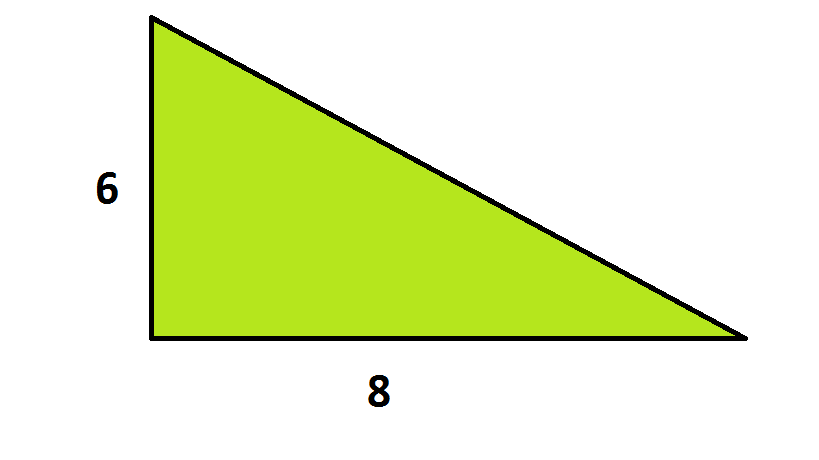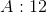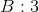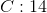What is a possible value for the length of the missing side?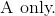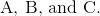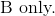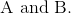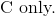Explanation:

For a triangle where the length of two sides,and, is the only information known, the third side,, is limited in the following matter: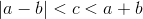For the triangle given: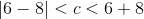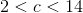.

Both choices A and B satisfy this criteria.

### Example Question #2 : How To Find The Length Of The Side Of An Acute / Obtuse Triangle

A triangle has sides of lengthsandQuantity A: The length of the missing side.

Quantity B:Quantity A is greater.

The relationship cannot be determined.

Quantity B is greater.

The two quantities are equal.

Quantity A is greater.

Explanation:

If two sides of a triangle are known and all angles are unknown, the length of the third side is limited by the difference and sum of the other two sides.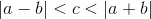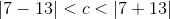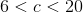The missing side must be greater than.

Quantity A is greater.

### Example Question #3 : How To Find The Length Of The Side Of An Acute / Obtuse Triangle

The lengths of two sides of a triangle areand.

Quantity A: The length of the missing side.

Quantity B:Quantity B is greater.

Quantity A is greater.

The relationship cannot be determined.

The two quantities are equal.

The relationship cannot be determined.

Explanation:

Seeing the sidesandmay bring to mind a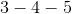triangle. However, we've been told nothing about the angles of the triangle. It could be right, or it could be obtuse or acute.

Since the angles are unknown, the side is bounded as follows: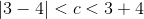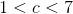There are plenty of potential lengths that fall above and below. The relationship cannot be determined.

### Example Question #4 : How To Find The Length Of The Side Of An Acute / Obtuse Triangle

A triangle has sidesandQuantity A: The length of the missing side.

Quantity B:Quantity A is greater.

The relationship cannot be determined.

The two quantities are equal.

Quantity B is greater.

Quantity B is greater.

Explanation:

If two sides of a triangle are known and the angles of the triangle are unknown, the length of the missing side is limited by the difference and sum of the other two sides.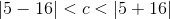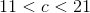For a triangle with sidesand, there is no way a side could be.

Quantity B is greater.

Tired of practice problems?

Try live online GRE prep today.